# Practical Exercise 12: THE MOON IN ACTION FOR THE THIRD TIME

a) Figure 6 shows the total Lunar eclipse. With the help of Figure 6 and times, estimate how many times the radius of the Earth’s shadow is larger than the radius of the Moon.

b) If the angular magnitude of the Sun is θ =  32', the radius of the Earth equals R = 6 378 km and the radius of the circular orbit is θ =32‘, the radius of the Earth equals R = 6 378 km and the radius of the circular orbit is a2 = 384 400 km, calculate with the help of the result in point a) the radius of the Moon. Figure 7 can help.Figure 6 Lunar EclipseFigure 7 Lunar Eclipse - situation analysis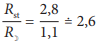b) The sketch shows the following: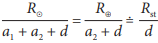Since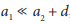, it is possible to write the left side of the first equation as, it is possible to write the left side of the first equation as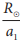, which is equal to, it is possible to write the left side of the first equation as, which is equal to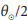. From the first equation we can simply express,it is possible to write the left side of the first equation as, which is equal to. From the first equation we can easily express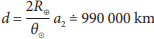. From the second equation we getit is possible to write the left side of the first equation as, which is equal to. From the first equation we can easily express. From the second equation we get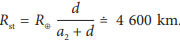.
We get the radius of the Moon as follows:, it is possible to write the left side of the first equation as, which is equal to. From the first equation we can easily express. From the second equation we get. We get the radius of the Moon as follows: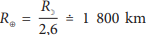. The actual radius of the Moon is 1737 km, so the result gives a reasonable estimate.